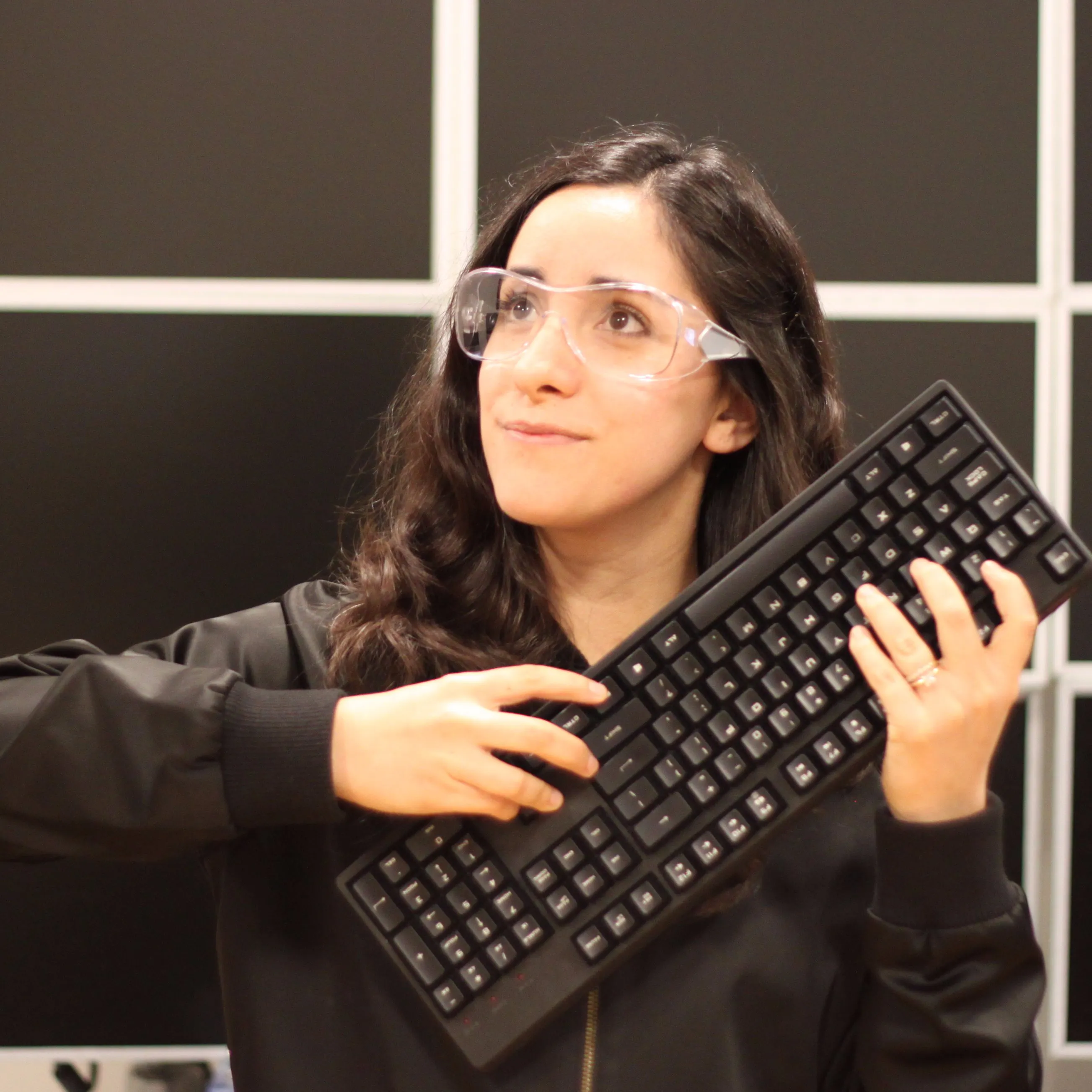# Halloween Build - Robosuit!

I like making robots, so I am going to be a robot.

BeginnerFull instructions provided4 hours2,740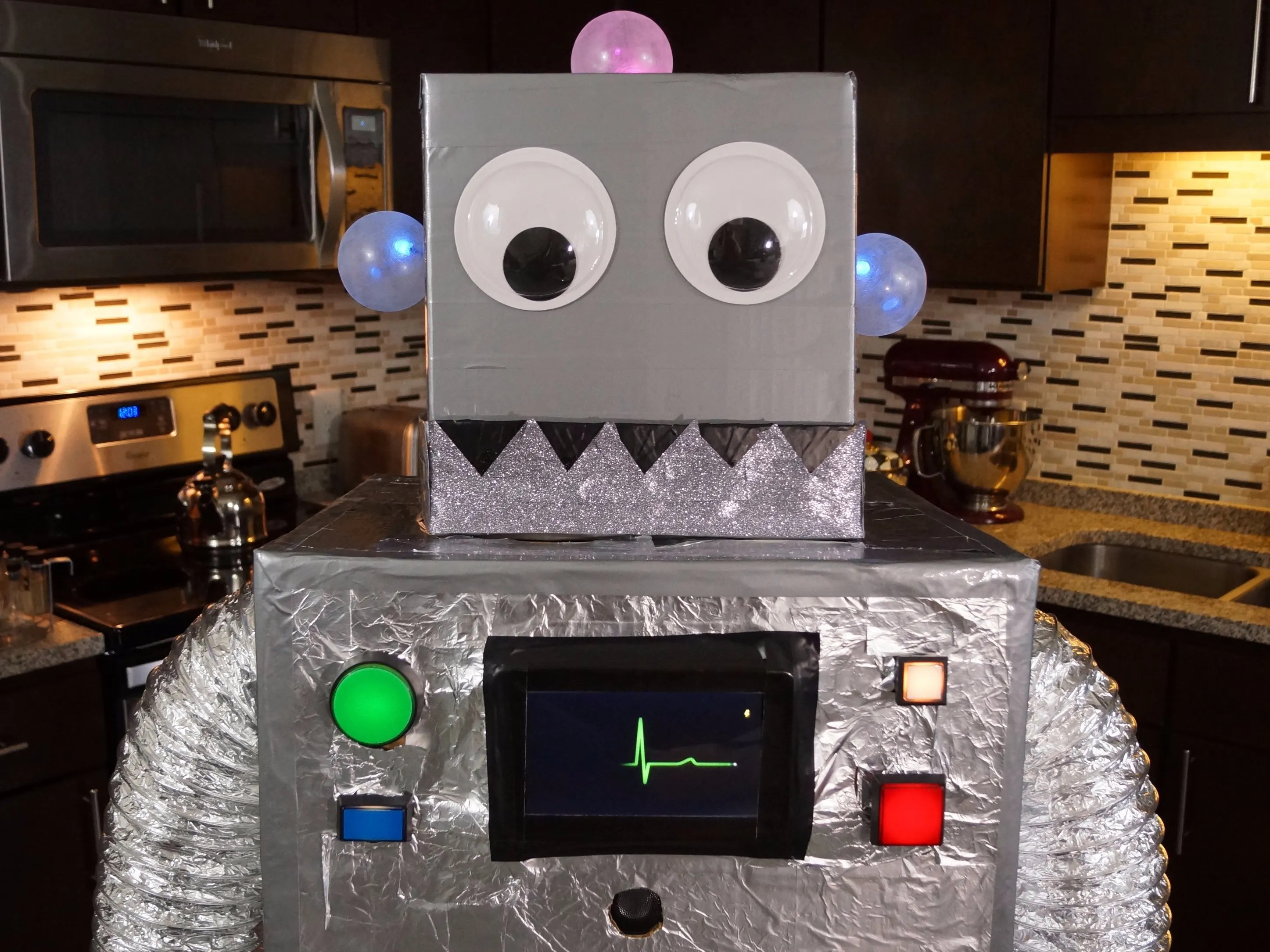## Things used in this project

### Hardware components

×1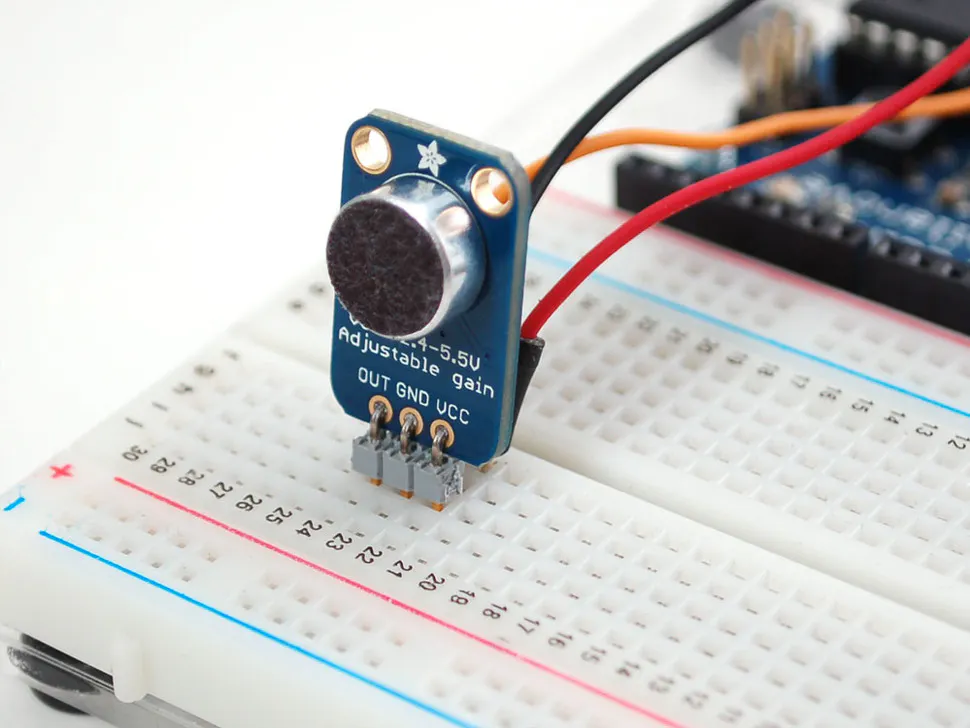×1Raspberry Pi 3 Model B
×1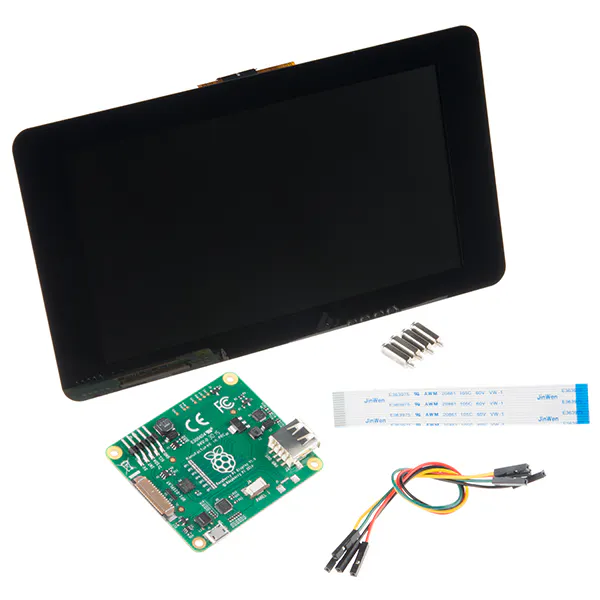Raspberry Pi Touch Display
×1
 10 mm RGB LEDs
×1
 Servo - TowerPro SG-5010 - 5010
×1

### Software apps and online servicesArduino IDE
 Raspberry Pi Raspian

## Schematics

### Arduino + Wave Shield + Microphone Amplifier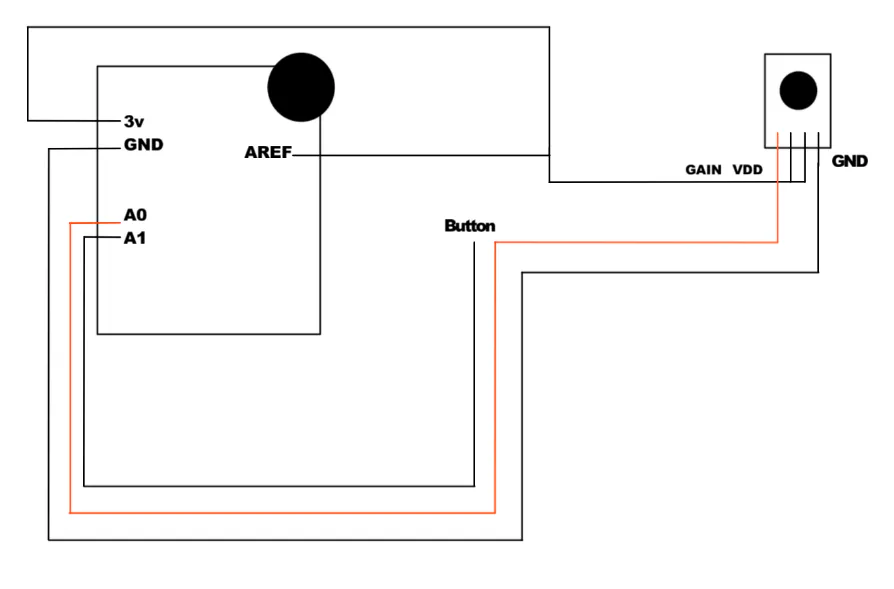### Arduino + Robot Jaw Servos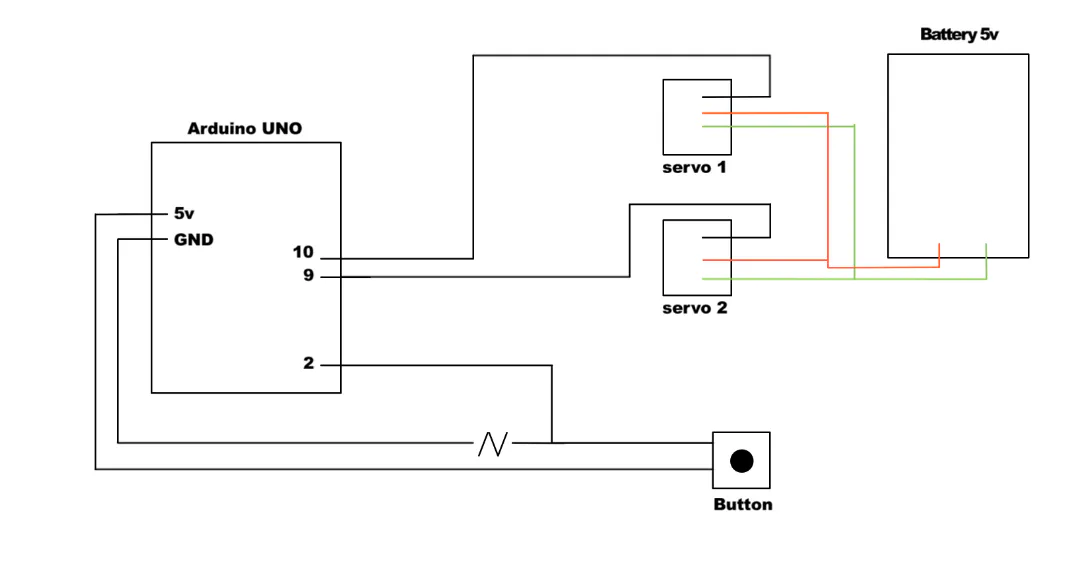### Arduino + RGB LEDs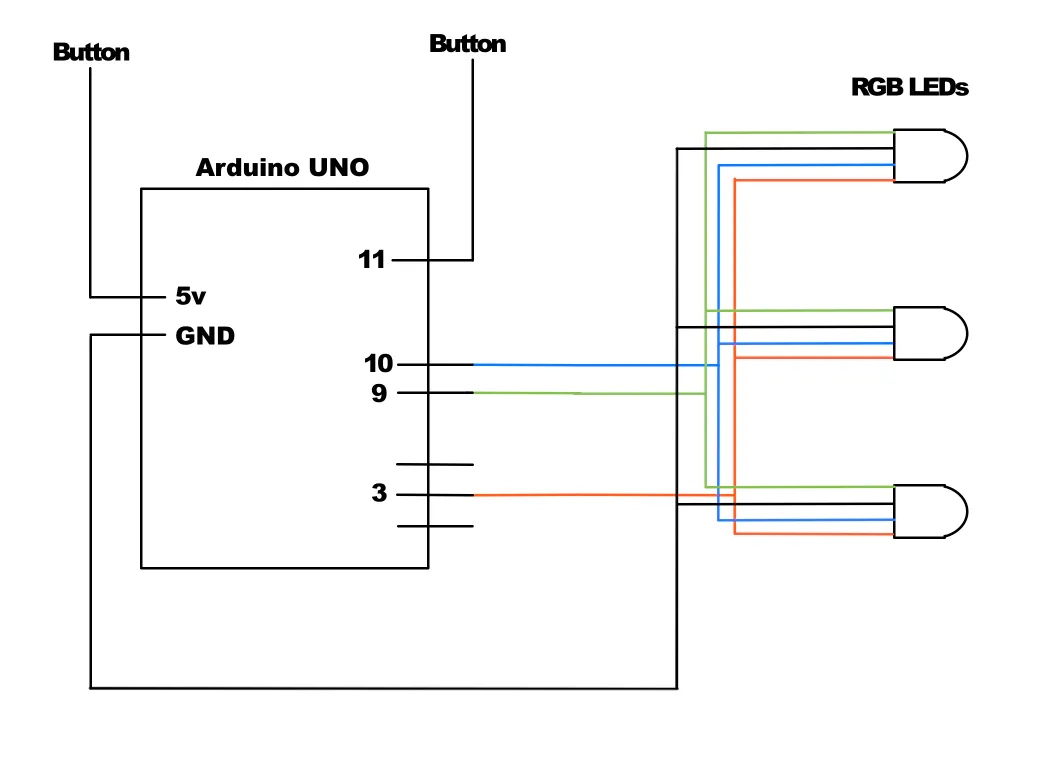### Raspberry Pi + Buttons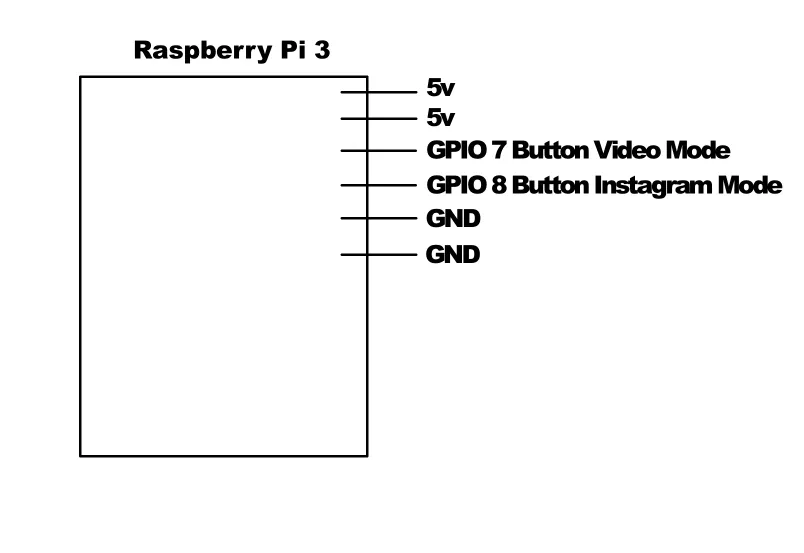## Code

### Heartbeat.py

Python
Python script to run the Instagram and video threads on the Pi. This script runs on the Pi and waits for a button signal.
```#This script has three threads, one for the Instagram function, one for the video player and one for the button.
#When the Instagram button is pressed, a blank video is played until the number of likes on the latest post increases.  When an increase is detected, a video of a electrocardiogram heartbeat plays.
#When the video button is pressed, the script plays a video.  Each press cycles through a predefined set of videos.

import requests
import json
import re
import time
import os
import sys
import subprocess
import RPi.GPIO as GPIO
from subprocess import Popen

users = ['estefanniegg']

buttonPin = 7
buttonPin2 = 8
GPIO.setmode(GPIO.BOARD)
GPIO.setup(buttonPin, GPIO.IN)
GPIO.setup(buttonPin2, GPIO.IN)

birthdayRobot = ("/home/pi/birthdayRobotMinute.mp4")
heartVideo= ("/home/pi/BeatMinute.mp4")
partyRobotVideo= ("/home/pi/partyRobotMinute.mp4")
chillingVideo = ("/home/pi/chillingRobot.mp4")

videoToPlay = 0

mode = 0
liveVideo = ("/home/pi/beat.mp4")
previous_like_count = 0
current_like_count = 0

global mode
while True:
condition.acquire()
buttonState = GPIO.input(buttonPin)
buttonState2 = GPIO.input(buttonPin2)

global videoToPlay
if buttonState != 0:
mode = 0
if videoToPlay < 4:
videoToPlay = videoToPlay + 1
else:
videoToPlay = 0
condition.notifyAll()
condition.release()

time.sleep(0.3)
elif buttonState2 != 0:
mode = 1
condition.notifyAll()
condition.release()
time.sleep(0.3)
else:
condition.release()

global mode
global liveVideo
global previous_like_count
global current_like_count

while True:
if mode == 1:
condition.acquire()
for user in users:
page = requests.get('https://www.instagram.com/' + user + '/')
# finding first post
text = page.text

# find where the posts start
finder_text_start = ('<script type="text/javascript">'
'window._sharedData = ')
finder_text_start_len = len(finder_text_start) - 1
finder_text_end = ';</script>'

all_data_start = text.find(finder_text_start)
all_data_end = text.find(finder_text_end, all_data_start + 1)
json_str = text[(all_data_start + finder_text_start_len + 1) \
: all_data_end]

# this puts posts into a list
media_by_tag = list(all_data['entry_data']['ProfilePage'] \
['user']['media']['nodes'])

# first post
print ('https://www.instagram.com/p/' + media_by_tag['code'] + '/')
postpage = requests.get('https://www.instagram.com/p/' + media_by_tag['code'] + '/')
postcontent = postpage.content
filtered = re.search(r"\"edge_media_preview_like\": {\"count\": \d+", postcontent)
likecount = re.search('(?<=t\": )\d+', filtered.group(0))
intlikecount = int(likecount.group(0))

current_like_count = intlikecount

os.system('killall omxplayer.bin')

if current_like_count > previous_like_count:
omxc = Popen(['omxplayer', '-b', liveVideo])
#time.sleep(4)
condition.wait(4)
else:
#time.sleep(1)
condition.wait(1)

print current_like_count
previous_like_count = current_like_count
condition.release()

global videoToPlay
global mode
while True:
condition.acquire()
if mode == 0:
os.system('killall omxplayer.bin')
if videoToPlay == 0:
omxc = Popen(['omxplayer', '-b', birthdayRobot])
elif videoToPlay == 1:
omxc = Popen(['omxplayer', '-b', heartVideo])
elif videoToPlay == 2:
omxc = Popen(['omxplayer', '-b', partyRobotVideo])
elif videoToPlay == 3:
omxc = Popen(['omxplayer', '-b', chillingVideo])
elif videoToPlay == 4:

condition.wait(60)
condition.release()

vt.start()
bt.start()
igt.start()
```

### RobotJaw.ino

C/C++
This code controls two servos, which opens and closes the robot jaw, depending on the input of a button. This is uploaded to the Arduino and starts up once it has power.
```/*This code controls two servos, which opens and closes the robot jaw, depending on the input of a button.  When the the button is held down, the mouth opens.  When the button is released, the mouth closes.*/

#include <Servo.h>

Servo servo1;  // create servo object to control a servo
Servo servo2;

int servoPos = 0;
const int buttonPin_1 = 2;
const int servoPin_1 = 9;
const int servoPin_2 = 10;

void setup()
{
Serial.begin(9600);
servo1.attach(servoPin_1);  // attaches the servo to pin
servo2.attach(servoPin_2);

pinMode(buttonPin_1, INPUT);
}

int mouthButton = 0;
bool opening = true;
int pos = 0;

void loop()
{

// mouth will open as button is held down and close as released
if (mouthButton == HIGH) // open mouth
{
if (servoPos < 45)
{
servo1.write(servoPos);
servo2.write(45 - servoPos);
// waits for the servo to reach the position
delay(2);
servoPos++;
}
}
else
{
if (servoPos > 0)
{
servo1.write(servoPos);
servo2.write(45 - servoPos);
delay(2);
servoPos--;
}
}
}
```

### RoboLights.ino

C/C++
A third Arduino for the RGB LEDs. This is uploaded to the Arduino and starts up once it has power.
```/* I used the third Arduino for the RGB LEDs, instead of combining them with the servo Arduino, because the multiple analogWrites to change the color of the LEDs caused timing conflicts with the servos.*/

const int buttonPin = 12;     // the number of the pushbutton pin
const int r1 = 3;     // the number of the pushbutton pin
const int g1 = 9;     // the number of the pushbutton pin
const int b1 = 10;     // the number of the pushbutton pin

//const int ledPin =  13;      // the number of the LED pin

void setup()
{
Serial.begin(9600);

pinMode(buttonPin, INPUT);

pinMode(r1, OUTPUT);
pinMode(g1, OUTPUT);
pinMode(b1, OUTPUT);
pinMode(ledPin, OUTPUT);
}

int ledButton = 0;
int ledState = 0;
bool opening = true;

void loop()
{
// light button pressed
if (ledButton == HIGH)
{
if (ledState == 0)
{
analogWrite(r1, 500);
analogWrite(g1, 10);
analogWrite(b1, 30);
ledState = 1;
}
else if (ledState == 1)
{
analogWrite(r1, 0);
analogWrite(g1, 500);
analogWrite(b1, 0);
ledState = 2;
}
else if (ledState == 2)
{
analogWrite(r1, 0);
analogWrite(g1, 0);
analogWrite(b1, 30);
ledState = 0;
}
// wait for button to release/avoid bounce

delay(100);
}
}
```

### RoboVoiceSounds.ino

C/C++
I used most of the adavoice_face code as a base for the voice modulation and sound playback. I modified the code to work with my button, and playback random sounds. This maxed out the capabilities of a single Arduino, so the other functions had to be on a different device.
```/*I used most of the adavoice_face code as a base for the voice modulation and sound playback.  I modified the code to work with my button, and playback  random sounds.  This maxed out the capabilities of a single Arduino, so the other functions had to be on a different device. */

#include <WaveHC.h>
#include <WaveUtil.h>

SdReader  card;  // This object holds the information for the card
FatVolume vol;   // This holds the information for the partition on the card
FatReader root;  // This holds the information for the volumes root directory
FatReader file;  // This object represent the WAV file for a pi digit or period
WaveHC    wave;  // This is the only wave (audio) object, -- we only play one at a time
#define error(msg) error_P(PSTR(msg))  // Macro allows error messages in flash memory

#define ADC_CHANNEL 0 // Microphone on Analog pin 0
#define BUTTON A1
#define DEBOUNCE 10  // Number of iterations before button 'takes'

// Wave shield DAC: digital pins 2, 3, 4, 5
#define DAC_CS_PORT    PORTD
#define DAC_CS         PORTD2
#define DAC_CLK_PORT   PORTD
#define DAC_CLK        PORTD3
#define DAC_DI_PORT    PORTD
#define DAC_DI         PORTD4
#define DAC_LATCH_PORT PORTD
#define DAC_LATCH      PORTD5

uint16_t in = 0, out = 0, xf = 0, nSamples; // Audio sample counters

// WaveHC didn't declare it's working buffers private or static,
// so we can be sneaky and borrow the same RAM for audio sampling!
extern uint8_t
buffer1[PLAYBUFFLEN],                   // Audio sample LSB
buffer2[PLAYBUFFLEN];                   // Audio sample MSB
#define MAX_SAMPLES (PLAYBUFFLEN - XFADE) // Remaining available audio samples

// Used for averaging all the audio samples currently in the buffer
uint8_t       oldsum = 0;
unsigned long newsum = 0L;

uint8_t
prev   = 255,                // Previous key reading (or 255 if none)
count  = 0;                  // Counter for button debouncing
#define DEBOUNCE 10            // Number of iterations before button 'takes'

const char *sound[] = { "breath" , "destroy", "zilla" , "burp" , "squirrel", "startup", "Ha" };

int buttonState = 0;

//////////////////////////////////// SETUP

void setup() {
uint8_t i;

Serial.begin(9600);

// The WaveHC library normally initializes the DAC pins...but only after
// an SD card is detected and a valid file is passed.  Need to init the
// pins manually here so that voice FX works even without a card.
pinMode(2, OUTPUT);    // Chip select
pinMode(3, OUTPUT);    // Serial clock
pinMode(4, OUTPUT);    // Serial data
pinMode(5, OUTPUT);    // Latch
digitalWrite(2, HIGH); // Set chip select high

// Init SD library, show root directory.  Note that errors are displayed
// but NOT regarded as fatal -- the program will continue with voice FX!
if(!card.init())             SerialPrint_P("Card init. failed!");
else if(!vol.init(card))     SerialPrint_P("No partition!");
else if(!root.openRoot(vol)) SerialPrint_P("Couldn't open dir");
else {
PgmPrintln("Files found:");
root.ls();
}

// Optional, but may make sampling and playback a little smoother:
// Disable Timer0 interrupt.  This means delay(), millis() etc. won't
// work.  Comment this out if you really, really need those functions.
TIMSK0 = 0;

// Set up Analog-to-Digital converter:
analogReference(EXTERNAL); // 3.3V to AREF

pinMode(BUTTON, INPUT);
// Whew! We got past the tough parts.
putstring_nl("Files found (* = fragmented):");

root.ls(LS_R | LS_FLAG_FRAGMENTED);

startPitchShift();     // and start the pitch-shift mode by default.
}

//////////////////////////////////// LOOP

void loop() {

int randomNumber = random(0, 7);

if(buttonInput == HIGH)
{
if(wave.isplaying)
{
wave.stop();
}
else
{
stopPitchShift();
}
playfile(randomNumber);
}

if(!wave.isplaying && !(TIMSK2 & _BV(TOIE2)))
{
startPitchShift();
PgmPrintln("microphone");
}
delay(20); // ~50 FPS
}

//////////////////////////////////// HELPERS

// Open and start playing a WAV file
void playfile(int idx) {
char filename;

(void)sprintf(filename,"%s.wav", sound[idx]);
//  Serial.print("File: ");
//  Serial.println(filename);

if(!file.open(root, filename)) {
PgmPrint("Couldn't open file ");
Serial.print(filename);
return;
}
if(!wave.create(file)) {
PgmPrintln("Not a valid WAV");
return;
}
wave.play();
}

//////////////////////////////////// PITCH-SHIFT CODE

void startPitchShift() {

// Read analog pitch setting before starting audio sampling:
int pitch = 100;
//Serial.print("Pitch: ");
//Serial.println(pitch);

// Right now the sketch just uses a fixed sound buffer length of
// 128 samples.  It may be the case that the buffer length should
// vary with pitch for better results...further experimentation
// is required here.
nSamples = 128;
//nSamples = F_CPU / 3200 / OCR2A; // ???
//if(nSamples > MAX_SAMPLES)      nSamples = MAX_SAMPLES;

memset(buffer1, 0, nSamples + XFADE); // Clear sample buffers
memset(buffer2, 2, nSamples + XFADE); // (set all samples to 512)

// WaveHC library already defines a Timer1 interrupt handler.  Since we
// want to use the stock library and not require a special fork, Timer2
// is used for a sample-playing interrupt here.  As it's only an 8-bit
// timer, a sizeable prescaler is used (32:1) to generate intervals
// spanning the desired range (~4.8 KHz to ~19 KHz, or +/- 1 octave
// from the sampling frequency).  This does limit the available number
// of speed 'steps' in between (about 79 total), but seems enough.
TCCR2A = _BV(WGM21) | _BV(WGM20); // Mode 7 (fast PWM), OC2 disconnected
TCCR2B = _BV(WGM22) | _BV(CS21) | _BV(CS20);  // 32:1 prescale
OCR2A  = map(pitch, 0, 1023,
F_CPU / 32 / (9615 / 2),  // Lowest pitch  = -1 octave
F_CPU / 32 / (9615 * 2)); // Highest pitch = +1 octave

// Start up ADC in free-run mode for audio sampling:
ADCSRB = 0;           // Free-run mode
_BV(ADPS0);         //  ...13 cycles/conversion = ~9615 Hz

TIMSK2 |= _BV(TOIE2); // Enable Timer2 overflow interrupt
sei();                // Enable interrupts
}

void stopPitchShift() {
TIMSK2 = 0;        // Disable Timer2 Interrupt
}

// Save old sample from 'in' position to xfade buffer:
buffer1[nSamples + xf] = buffer1[in];
buffer2[nSamples + xf] = buffer2[in];
if(++xf >= XFADE) xf = 0;

// Store new value in sample buffers:

newsum += abs((((int)buffer2[in] << 8) | buffer1[in]) - 512);

if(++in >= nSamples) {
in     = 0;
oldsum = (uint8_t)((newsum / nSamples) >> 1); // 0-255
newsum = 0L;
}
}

ISR(TIMER2_OVF_vect) { // Playback interrupt
uint16_t s;
uint8_t  w, inv, hi, lo, bit;
int      o2, i2, pos;

// Cross fade around circular buffer 'seam'.
if((o2 = (int)out) == (i2 = (int)in)) {
// Sample positions coincide.  Use cross-fade buffer data directly.
pos = nSamples + xf;
hi = (buffer2[pos] << 2) | (buffer1[pos] >> 6); // Expand 10-bit data
lo = (buffer1[pos] << 2) |  buffer2[pos];       // to 12 bits
} if((o2 < i2) && (o2 > (i2 - XFADE))) {
// Output sample is close to end of input samples.  Cross-fade to
// avoid click.  The shift operations here assume that XFADE is 16;
// will need adjustment if that changes.
w   = in - out;  // Weight of sample (1-n)
pos = nSamples + ((inv + xf) % XFADE);
s   = ((buffer2[out] << 8) | buffer1[out]) * w +
((buffer2[pos] << 8) | buffer1[pos]) * inv;
hi = s >> 10; // Shift 14 bit result
lo = s >> 2;  // down to 12 bits
} else if (o2 > (i2 + nSamples - XFADE)) {
w   = in + nSamples - out;
pos = nSamples + ((inv + xf) % XFADE);
s   = ((buffer2[out] << 8) | buffer1[out]) * w +
((buffer2[pos] << 8) | buffer1[pos]) * inv;
hi = s >> 10; // Shift 14 bit result
lo = s >> 2;  // down to 12 bits
} else {
// Input and output counters don't coincide -- just use sample directly.
hi = (buffer2[out] << 2) | (buffer1[out] >> 6); // Expand 10-bit data
lo = (buffer1[out] << 2) |  buffer2[out];       // to 12 bits
}

// Might be possible to tweak 'hi' and 'lo' at this point to achieve
// different voice modulations -- robot effect, etc.?

DAC_CS_PORT &= ~_BV(DAC_CS); // Select DAC
// Clock out 4 bits DAC config (not in loop because it's constant)
DAC_DI_PORT  &= ~_BV(DAC_DI); // 0 = Select DAC A, unbuffered
DAC_CLK_PORT |=  _BV(DAC_CLK); DAC_CLK_PORT &= ~_BV(DAC_CLK);
DAC_CLK_PORT |=  _BV(DAC_CLK); DAC_CLK_PORT &= ~_BV(DAC_CLK);
DAC_DI_PORT  |=  _BV(DAC_DI); // 1X gain, enable = 1
DAC_CLK_PORT |=  _BV(DAC_CLK); DAC_CLK_PORT &= ~_BV(DAC_CLK);
DAC_CLK_PORT |=  _BV(DAC_CLK); DAC_CLK_PORT &= ~_BV(DAC_CLK);
for(bit=0x08; bit; bit>>=1) { // Clock out first 4 bits of data
if(hi & bit) DAC_DI_PORT |=  _BV(DAC_DI);
else         DAC_DI_PORT &= ~_BV(DAC_DI);
DAC_CLK_PORT |=  _BV(DAC_CLK); DAC_CLK_PORT &= ~_BV(DAC_CLK);
}
for(bit=0x80; bit; bit>>=1) { // Clock out last 8 bits of data
if(lo & bit) DAC_DI_PORT |=  _BV(DAC_DI);
else         DAC_DI_PORT &= ~_BV(DAC_DI);
DAC_CLK_PORT |=  _BV(DAC_CLK); DAC_CLK_PORT &= ~_BV(DAC_CLK);
}
DAC_CS_PORT    |=  _BV(DAC_CS);    // Unselect DAC

if(++out >= nSamples) out = 0;
}
```

## Credits

### Estefannie Explains It All

0 projects • 80 followers
I make things in my kitchen and put it on YouTube. Sometimes I also make computer science tutorials.